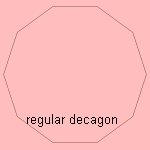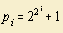# (simple) polygon

## line

last updated: 2006-04-06

A polygon 1) is a closed curve, composed of straight line parts.

In the Netherlands, in the sixties the 'Polygoon' news-reel showed us news from all over the world in the cinema.

Another page is dedicated to the (nonsimple) star polygons.An equilateral polygon has sides of equal length, an equiangular polygon has equal angles. Only for a triangle the equilateral and equiangular version gives the same figure. The two qualities together make a regular polygon. The more sides the polygon has, the more it approximates a circle. The genius mathematician Gauss showed - in the age of 18 - which regular polygons can be constructed with a pair of compasses and a ruler 2). For example the regular heptadecagon has this quality.

The polygons have been given own names, derived from the Greek numerals:

 number of sides name of polygon 1 henagon or monogon 2 digon 3 triangle 4 quadrilateral 5 pentagon 6 hexagon 7 heptagon 8 octagon 9 enneagon 10 decagon 11 hendecagon 12 dodecagon 13 tridecagon or triskaidecagon 14 tetradecagon 15 pentadecagon, pentakaidecagon or quindecagon 16 hexadecagon or hexakaidecagon 17 heptadecagon, heptakaidecagon, or septadecagon 18 octadecagon or octakaidecagon 19 enneadecagon, enneakaidecagon, or nonadecagon 20 icosagon 21 icosikaihenagon or henicosagon 22 icosikaidigon or docosagon 23 icosikaitrigon or tricosagon 24 icosikaitetragon or tetracosagon 25 icosikaipentagon or pentacosagon 26 icosikaihexagon or hexacosagon 27 icosikaiheptagon or heptacosagon 28 icosikaioctagon or octacosagon 29 icosikaienneagon, enneacosagon or nonacosagon 30 triacontagon 31 triacontakaihenagon or henitriacontagon 32 triacontakaidigon or dotriacontagon 40 tetracontagon 50 pentacontagon 60 hexacontagon 70 heptacontagon 80 octacontagon 90 enneacontagon or nonacontagon 100 hectagon 1000 chiliagon 10000 myriagon 1000000 hecatommyriagon or hekatommyriagon

The first two polygons mentioned are one-dimensional, they consist both of just one side.

Some polygons derive their qualities in relation to their position according another curve:

• a cyclic polygon is a polygon where the sides are chords of a given circle
• for a tangent polygon the sides are tangent to a given curve (in most cases a circle)
• the circumscribed polygon and the inscribed polygon: when on each side of a polygon there lies a vertex of a second polygon, we call the outer polygon a circumscribed polygon, and the inner polygon an inscribed polygon

A so-called control polygon is used to define a curve by points near the curve, not by points on the curve.

In the field of Roman ancient history the so called Thijssen polygons are in use: these polygons have been constructed around Roman district capitals, to approximate the districts' boundaries.

I dedicated a separate page to the family of stars.

You can also imagine polygons build of circle arcs, these are curvilinear polygons.

notes

1) Poly (Gr.) = many, goonia (Gr.) = angle.

2)  When n is the number of sides, then for be able to construct by a pair of compasses, n has to obey:where pi is the Fermat Number for which: